# AP Physics C: Mechanics : Calculating Motion in One Dimension

## Example Questions

### Example Question #12 : Motion

A man runs with a velocity as described by the function below.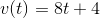How far does he travel in 1 minute?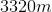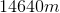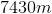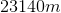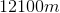Explanation:

Distance is given by the integral of a velocity function. For this question, we will need to integrate over the interval of 0s to 60s.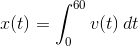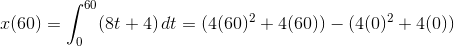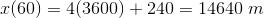### Example Question #13 : Motion

A man runs with a velocity described by the function below.What is the function for his acceleration?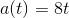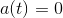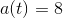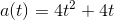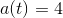Explanation:

The function for acceleration is the derivative of the function for velocity.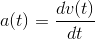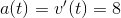### Example Question #14 : Motion

A particle traveling in a straight line accelerates uniformly from rest to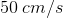in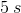and then continues at constant speed for an additional for an additional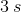. What is the total distance traveled by the particle during the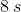?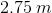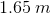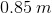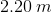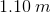Explanation:

First off we have to convertto meters per second.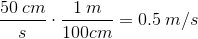Next we have to calculate the distance the object traveled the first 5 seconds, when it was starting from rest. We are given time, initial speed to be. The acceleration of the object at this time can be calculated using: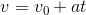, substituting the values, we get: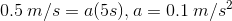Next, we use the distance equation to find the distance in the first 5 seconds: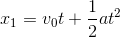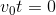because the initial speed is.

If we plug in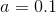,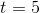, we get: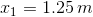Next we have to find the distance the the object travels at constant speed for 3 seconds. We can use the equation: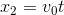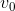in this case is not equal to, it is equal to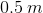and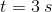Plugging in the equation, we get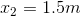Adding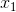and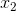we get the total distance to be